# AP Calculus AB Practice Test 38

### Test Information9 questions18 minutes

Calculator Disallowed

1. Use the graph below, consisting of two line segments and a quarter-circle. The graphshows the velocity of an object during a 6-second interval.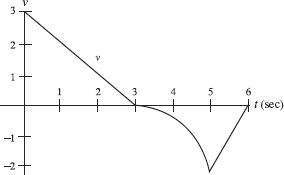For how many values of t in the interval 0 < t < 6 is the acceleration undefined?

2. Use the graph below, consisting of two line segments and a quarter-circle. The graphshows the velocity of an object during a 6-second interval.During what time interval (in sec) is the speed increasing?

3. Use the graph below, consisting of two line segments and a quarter-circle. The graphshows the velocity of an object during a 6-second interval.What is the average acceleration (in units/sec2) during the first 5 seconds?

4. The curve of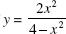has

5. Suppose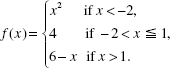Which statement is true?

6. The function f (x) = x5 + 3x - 2 passes through the point (1, 2). Let f -1 denote the inverse of f. Then (f -1) (2) equals

7.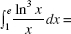8. Which of the following statements is (are) true about the graph of y = ln (4 + x2)?

I. It is symmetric to the y-axis.

II. It has a local minimum at x = 0.

III. It has inflection points at x = ±2.

9. Let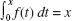sin πx. Then f (3) =